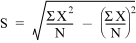# 17.10 - Defining What to Store in the Storage Area - Advanced SQL Engine - Teradata Database

## Teradata Vantage™ - SQL External Routine Programming

Product
Release Number
17.10
Release Date
July 2021
Content Type
Programming Reference
Publication ID
B035-1147-171K
Language
English (United States)

The type of intermediate results that an aggregate UDF needs to save depends on the type of calculation that the UDF performs and whether the method wants to represent the intermediate results as a byte array or as an object.

Consider a standard deviation function STD_DEV(x) that uses the following equation:Based on the calculation, the UDF needs to store the following:
• N
• sum(X 2)
• sum(X)

Here is a Java class called agr_storage with fields that match the necessary intermediate values:

```class agr_storage implements Serializable{
double count;
double x_sq;
double x_sum;

public agr_storage(double a, double b, double c){
count = a;
x_sq = b;
x_sum = c;
}
}```

Alternatively, especially when better performance is required, the method that implements the aggregate UDF can use a ByteBuffer for the intermediate results and use the putDouble() and getDouble() methods on the ByteBuffer for the necessary intermediate values.